# Migrate QRadar detection rules to Microsoft Sentinel

## Identify and migrate rules

Microsoft Sentinel uses machine learning analytics to create high-fidelity and actionable incidents, and some of your existing detections may be redundant in Microsoft Sentinel. Therefore, don't migrate all of your detection and analytics rules blindly. Review these considerations as you identify your existing detection rules.

• Make sure to select use cases that justify rule migration, considering business priority and efficiency.
• Check that you understand Microsoft Sentinel rule types.
• Check that you understand the rule terminology.
• Review any rules that haven't triggered any alerts in the past 6-12 months, and determine whether they're still relevant.
• Eliminate low-level threats or alerts that you routinely ignore.
• Use existing functionality, and check whether Microsoft Sentinel’s built-in analytics rules might address your current use cases. Because Microsoft Sentinel uses machine learning analytics to produce high-fidelity and actionable incidents, it’s likely that some of your existing detections won’t be required anymore.
• Confirm connected data sources and review your data connection methods. Revisit data collection conversations to ensure data depth and breadth across the use cases you plan to detect.
• Explore community resources such as the SOC Prime Threat Detection Marketplace to check whether your rules are available.
• Consider whether an online query converter such as Uncoder.io might work for your rules.
• If rules aren’t available or can’t be converted, they need to be created manually, using a KQL query. Review the rules mapping to create new queries.

To migrate your analytics rules to Microsoft Sentinel:

1. Verify that you have a testing system in place for each rule you want to migrate.

1. Prepare a validation process for your migrated rules, including full test scenarios and scripts.

2. Ensure that your team has useful resources to test your migrated rules.

3. Confirm that you have any required data sources connected, and review your data connection methods.

2. Verify whether your detections are available as built-in templates in Microsoft Sentinel:

• If the built-in rules are sufficient, use built-in rule templates to create rules for your own workspace.

In Microsoft Sentinel, go to the Configuration > Analytics > Rule templates tab, and create and update each relevant analytics rule.

• If you have detections that aren't covered by Microsoft Sentinel's built-in rules, try an online query converter, such as Uncoder.io to convert your queries to KQL.

Identify the trigger condition and rule action, and then construct and review your KQL query.

• If neither the built-in rules nor an online rule converter is sufficient, you'll need to create the rule manually. In such cases, use the following steps to start creating your rule:

1. Identify the data sources you want to use in your rule. You'll want to create a mapping table between data sources and data tables in Microsoft Sentinel to identify the tables you want to query.

2. Identify any attributes, fields, or entities in your data that you want to use in your rules.

3. Identify your rule criteria and logic. At this stage, you may want to use rule templates as samples for how to construct your KQL queries.

Consider filters, correlation rules, active lists, reference sets, watchlists, detection anomalies, aggregations, and so on. You might use references provided by your legacy SIEM to understand how to best map your query syntax.

4. Identify the trigger condition and rule action, and then construct and review your KQL query. When reviewing your query, consider KQL optimization guidance resources.

3. Test the rule with each of your relevant use cases. If it doesn't provide expected results, you may want to review the KQL and test it again.

4. When you're satisfied, you can consider the rule migrated. Create a playbook for your rule action as needed. For more information, see Automate threat response with playbooks in Microsoft Sentinel.

## Compare rule terminology

This table helps you to clarify the concept of a rule in Microsoft Sentinel compared to QRadar.

Rule type • Events
• Flow
• Common
• Offense
• Anomaly detection rules
• Scheduled query
• Fusion
• Microsoft Security
• Machine Learning (ML) Behavior Analytics
Criteria Define in test condition Define in KQL
Trigger condition Define in rule Threshold: Number of query results
Action • Create offense
• Dispatch new event
• Add to reference set or data
• And more
• Integrates with Logic Apps

## Map and compare rule samples

Use these samples to compare and map rules from QRadar to Microsoft Sentinel in various scenarios.

Rule Syntax Sample detection rule (QRadar) Sample KQL query Resources
Common property tests QRadar syntax Regular expression example
AQL filter query example
equals/not equals example
Regular expression example
AQL filter query example
equals/not equals example
• Regular expression: matches regex
• AQL filter query: string operators
• equals/not equals: String operators
Date/time tests QRadar syntax Selected day of the month example
Selected day of the week example
after/before/at example
Selected day of the month example
Selected day of the week example
after/before/at example
Date and time operators
• Selected day of the month: dayofmonth()
• Selected day of the week: dayofweek()
• after/before/at: format_datetime()
Event property tests QRadar syntax IP protocol example
IP protocol example
• IP protocol: String operators
Functions: counters QRadar syntax Event property and time example Event property and time example summarize
Functions: negative conditions QRadar syntax Negative conditions example Negative conditions example join()
String operators
Numerical operators
Functions: simple QRadar syntax Simple conditions example Simple conditions example or
IP/port tests QRadar syntax Source port example
Source IP example
Source port example
Source IP example
Log source tests QRadar syntax Log source example Log source example

### Common property tests syntax

Here's the QRadar syntax for a common property tests rule.### Common property tests: Regular expression example (QRadar)

Here's the syntax for a sample QRadar common property tests rule that uses a regular expression:

``````when any of <these properties> match <this regular expression>
``````

Here's the sample rule in QRadar.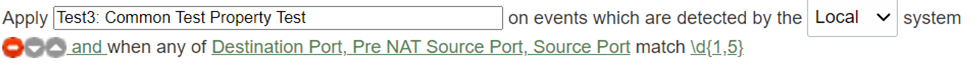### Common property tests: Regular expression example (KQL)

Here's the common property tests rule with a regular expression in KQL.

``````CommonSecurityLog
| where tostring(SourcePort) matches regex @"\d{1,5}" or tostring(DestinationPort) matches regex @"\d{1,5}"
``````

### Common property tests: AQL filter query example (QRadar)

Here's the syntax for a sample QRadar common property tests rule that uses an AQL filter query.

``````when the event matches <this> AQL filter query
``````

Here's the sample rule in QRadar.### Common property tests: AQL filter query example (KQL)

Here's the common property tests rule with an AQL filter query in KQL.

``````CommonSecurityLog
| where SourceIP == '10.1.1.10'
``````

### Common property tests: equals/not equals example (QRadar)

Here's the syntax for a sample QRadar common property tests rule that uses the `equals` or `not equals` operator.

``````and when <this property> <equals/not equals> <this property>
``````

Here's the sample rule in QRadar.### Common property tests: equals/not equals example (KQL)

Here's the common property tests rule with the `equals` or `not equals` operator in KQL.

``````CommonSecurityLog
| where SourceIP == DestinationIP
``````

### Date/time tests syntax

Here's the QRadar syntax for a date/time tests rule.### Date/time tests: Selected day of the month example (QRadar)

Here's the syntax for a sample QRadar date/time tests rule that uses a selected day of the month.

``````and when the event(s) occur <on/after/before> the <selected> day of the month
``````

Here's the sample rule in QRadar.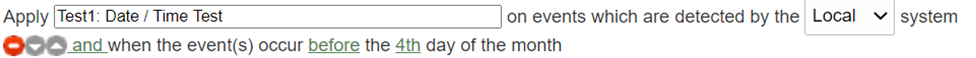### Date/time tests: Selected day of the month example (KQL)

Here's the date/time tests rule with a selected day of the month in KQL.

``````SecurityEvent
| where dayofmonth(TimeGenerated) < 4
``````

### Date/time tests: Selected day of the week example (QRadar)

Here's the syntax for a sample QRadar date/time tests rule that uses a selected day of the week:

``````and when the event(s) occur on any of <these days of the week{Monday, Tuesday, Wednesday, Thursday, Friday, Saturday, Sunday}>
``````

Here's the sample rule in QRadar.### Date/time tests: Selected day of the week example (KQL)

Here's the date/time tests rule with a selected day of the week in KQL.

``````SecurityEvent
| where dayofweek(TimeGenerated) between (3d .. 5d)
``````

### Date/time tests: after/before/at example (QRadar)

Here's the syntax for a sample QRadar date/time tests rule that uses the `after`, `before`, or `at` operator.

``````and when the event(s) occur <after/before/at> <this time{12.00AM, 12.05AM, ...11.50PM, 11.55PM}>
``````

Here's the sample rule in QRadar.### Date/time tests: after/before/at example (KQL)

Here's the date/time tests rule that uses the `after`, `before`, or `at` operator in KQL.

``````SecurityEvent
| where format_datetime(TimeGenerated,'HH:mm')=="23:55"
``````

`TimeGenerated` is in UTC/GMT.

### Event property tests syntax

Here's the QRadar syntax for an event property tests rule.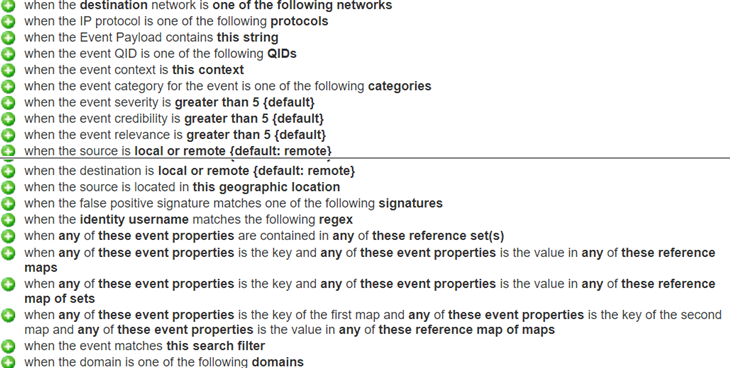### Event property tests: IP protocol example (QRadar)

Here's the syntax for a sample QRadar event property tests rule that uses an IP protocol.

``````and when the IP protocol is one of the following <protocols>
``````

Here's the sample rule in QRadar.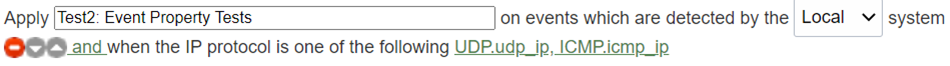### Event property tests: IP protocol example (KQL)

``````CommonSecurityLog
| where Protocol in ("UDP","ICMP")
``````

Here's the syntax for a sample QRadar event property tests rule that uses an `Event Payload` string value.

``````and when the Event Payload contains <this string>
``````

Here's the sample rule in QRadar.### Event property tests: Event Payload string example (KQL)

``````CommonSecurityLog
| where DeviceVendor has "Palo Alto"

search "Palo Alto"
``````

To optimize performance, avoid using the `search` command if you already know the table name.

### Functions: counters syntax

Here's the QRadar syntax for a functions rule that uses counters.### Counters: Event property and time example (QRadar)

Here's the syntax for a sample QRadar functions rule that uses a defined number of event properties in a defined number of minutes.

``````and when at least <this many> events are seen with the same <event properties> in <this many> <minutes>
``````

Here's the sample rule in QRadar.### Counters: Event property and time example (KQL)

``````CommonSecurityLog
| summarize Count = count() by SourceIP, DestinationIP
| where Count >= 5
``````

### Functions: negative conditions syntax

Here's the QRadar syntax for a functions rule that uses negative conditions.Here's the syntax for a sample QRadar functions rule that uses negative conditions.

``````and when none of <these rules> match in <this many> <minutes> after <these rules> match with the same <event properties>
``````

Here are two defined rules in QRadar. The negative conditions will be based on these rules.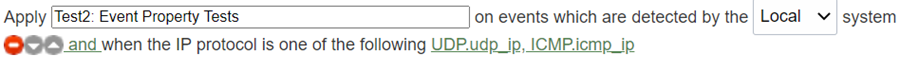Here's a sample of the negative conditions rule based on the rules above.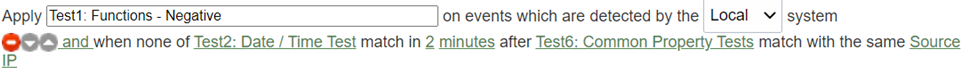### Negative conditions example (KQL)

``````let spanoftime = 10m;
let Test2 = (
CommonSecurityLog
| where Protocol !in ("UDP","ICMP")
| where TimeGenerated > ago(spanoftime)
);
let Test6 = (
CommonSecurityLog
| where SourceIP == DestinationIP
);
Test2
| join kind=rightanti Test6 on \$left. SourceIP == \$right. SourceIP and \$left. Protocol ==\$right. Protocol
``````

### Functions: simple conditions syntax

Here's the QRadar syntax for a functions rule that uses simple conditions.Here's the syntax for a sample QRadar functions rule that uses simple conditions.

``````and when an event matches <any|all> of the following <rules>
``````

Here's the sample rule in QRadar.### Simple conditions example (KQL)

``````CommonSecurityLog
| where Protocol !in ("UDP","ICMP") or SourceIP == DestinationIP
``````

### IP/port tests syntax

Here's the QRadar syntax for an IP/port tests rule.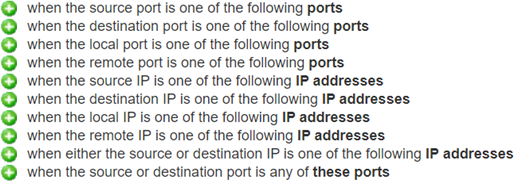### IP/port tests: Source port example (QRadar)

Here's the syntax for a sample QRadar rule specifying a source port.

``````and when the source port is one of the following <ports>
``````

Here's the sample rule in QRadar.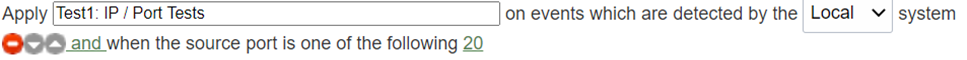### IP/port tests: Source port example (KQL)

``````CommonSecurityLog
| where SourcePort == 20
``````

### IP/port tests: Source IP example (QRadar)

Here's the syntax for a sample QRadar rule specifying a source IP.

``````and when the source IP is one of the following <IP addresses>
``````

Here's the sample rule in QRadar.### IP/port tests: Source IP example (KQL)

``````CommonSecurityLog
| where SourceIP in (“10.1.1.1”,”10.2.2.2”)
``````

### Log source tests syntax

Here's the QRadar syntax for a log source tests rule.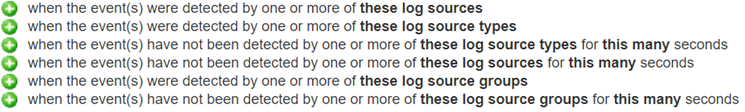Here's the syntax for a sample QRadar rule specifying log sources.

``````and when the event(s) were detected by one or more of these <log source types>
``````

Here's the sample rule in QRadar.#### Log source example (KQL)

``````OfficeActivity